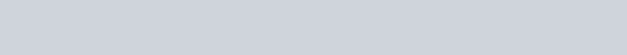# Python enumerate()

Filed Under: Python

In this tutorial, we are going to learn about Python enumerate() function. This is one of the built-in functions in Python.

## Python enumerate()

Python enumerate takes a sequence, and then make each element of the sequence into a tuple. The first element of the tuple is the index number. And the second element of the tuple is the value of the sequence.

So, in short, we can say that enumerate adds a counter with the element of a sequence. The basic syntax of python enumerate function is given below.

1. `enumerate(sequence) :` This enumerate function make a enumerate object where index starts from 0.
2. `enumerate(sequence, i):` This makes an enumerate object where the index starts from i.

### Python Enumerate List

In this section, we will see an example to create an enumerate object from a list or any other sequence. In the previous section, we learned about enumerate function which converts a sequence to enumerate object. Let’s see the following example.

``````
# initialize a list of list
data = ['Love', 'Hate', 'Death', 123, ['Alice', 'Bob', 'Trudy']]

# print the type of variable 'data'
print('The type of data is :', type(data))  # output is 'list'

data = enumerate(data)
# again, print the type of variable 'data'
print('The type of data is now :', type(data))  # output is 'enumerate'
``````

The output of the following code will be### Accessing Python Enumerate Object

We can access the enumerate object. We can use for loop to access the enumerate object. Or, we can convert the enumerate object to a list object.

Then we can traverse the list like we did in our python list tutorial. Let’s have a look to the following example to understand this.

``````
# initialize a list of list
data = ['Love', 'Hate', 'Death', 123, ['Alice', 'Bob', 'Trudy']]
# make an enumerate object
enumObject = enumerate(data)

# access the enumerate object using loop
for element in enumObject:
print(element)

print('\nStart index is changed to 100:')
# change the start index of the list to 100
enumObject = enumerate(data, 100)

# access the enumerate object using loop
for element in enumObject:
print(element)

``````

Output:

``````
(0, 'Love')
(1, 'Hate')
(2, 'Death')
(3, 123)
(4, ['Alice', 'Bob', 'Trudy'])

Start index is changed to 100:
(100, 'Love')
(101, 'Hate')
(102, 'Death')
(103, 123)
(104, ['Alice', 'Bob', 'Trudy'])
``````

So, that’s the basics of Python enumerate function. Usually you may not need it always but it’s not bad to know about new things. For any query regarding this topic, please use the comment box. Happy coding. 🙂

You can checkout complete python script and more Python examples from our GitHub Repository.

Reference: Official Documentation

close
Generic selectors
Exact matches only
Search in title
Search in content